# GMAT Math : Circles

## Example Questions

### Example Question #1 : Sectors

Walder is at his cousin's wedding preparing to eat a slice of pie.

I) Walder's slice has a radius of half a meter

II) Walder's slice is 5% of the total pie

What is the central angle of Walder's slice?

Both statements are needed to answer the question.

Statement I is sufficient to answer the question, but Statement II is not sufficient to answer the question.

Either statement is sufficient to answer the question.

Statement II is sufficient to answer the question, but Statement I is not sufficient to answer the question.

Statement II is sufficient to answer the question, but Statement I is not sufficient to answer the question.

Explanation:

The central angle of a sector, in this case represented by the slice of pie, can be thought of as a percentage of the whole circle. Circles have 360 degrees.

Statement I gives us the radius of the circle. We could find the diameter, area, or circumference with this, but not the central angle of that slice.

Statement II gives us the percentage of the whole circle that the slice represents, 5%. We can use this to find the number of degrees in the central angle of the slice, because it will just be 5% of 360.

Thus, Statement II is sufficient, but Statement I is not.

To recap:

Walder is at his cousin's wedding preparing to eat a slice of pie.

I) Walder's slice has a radius of half a meter

II) Walder's slice is 5% of the total pie

What is the central angle of Walder's slice?

Use Statement II to find the angle. The angle must be 5% of 360: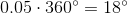### Example Question #1 : Sectors

Alex decided to order a circular pizza. Find the angle that represents the slice of pizza he ate.

II) The slice Alex ate represented one-fifth of the total pizza.

Both statements are needed to answer the question.

Statement I is sufficient to answer the question, but statement II is not sufficient to answer the question.

Statement II is sufficient to answer the question, but statement I is not sufficient to answer the question.

Either statement is sufficient to answer the question.

Statement II is sufficient to answer the question, but statement I is not sufficient to answer the question.

Explanation:

To find the angle of a sector (in this case, that represented by the slice of pizza), we need to know with how much of the circle we are dealing.

Statement I gives us the radius of the circle. This is helpful for a lot of other things, but not finding our central angle.

Statement II tells us what portion of the pizza we are concerned with. We can multiply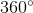by one-fifth to get the correct answer.

Using Statement II if the slice is one-fifth of the total pizza, then we can do the following to find the answer: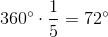Thus, Statement II is sufficient, but Statement I is not.

### Example Question #5 : Sectors

Find the angle for the percentage of a circle.

Statement 1:  A circle diameter is 5.

Statement 2:  The sector length is.

Statement 1 ALONE is sufficient, but Statement 2 ALONE is not sufficient to answer the question.

EACH statement ALONE is sufficient.

BOTH statements taken TOGETHER are sufficient to answer the question, but neither statement ALONE is sufficient.

BOTH statements TOGETHER are NOT sufficient, and additional data is needed to answer the question.

Statement 2 ALONE is sufficient, but Statement 1 ALONE is not sufficient to answer the question.

Statement 1 ALONE is sufficient, but Statement 2 ALONE is not sufficient to answer the question.

Explanation:

The question asks to solve the angle of a percentage of a circle.

Statement 1):  A circle diameter is 5.

Statement 1) is sufficient to solve for the angle of the circle because the statement itself provides that the shape is a full circle, 360 degrees, and is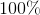of the circular sector.

Statement 2):  The sector length is.

Statement 2) does not have sufficient information to solve for the angle.  The precentage of the circle is not provided and we do not know how much of the circle will have a sector length of.  We also cannot assume that the sector is a full circle to make any further conclusions.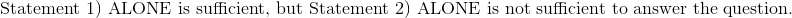### Example Question #1 : Sectors

Dirk bought a sticker to cover part of the base of his scuba tank. The sticker and the base have the same radius. Given the following, find the percentage of the base that the sticker covers.

I) The central angle of the sticker is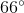II) The base of the tank has a radius of 5 inches.

Statement II is sufficient to answer the question, but Statement I is not sufficient to answer the question.

Either statement is sufficient to answer the question.

Both statements are needed to answer the question.

Statement I is sufficient to answer the question, but Statement II is not sufficient to answer the question.

Statement I is sufficient to answer the question, but Statement II is not sufficient to answer the question.

Explanation:

To find the percentage that the sticker covers, we need to know what portion of the circle it covers. We could find this either with the area of the circle or the area of the sector, but we don't have a good way to find the area of the sector.

Statement I gives us the degree measurement of the sticker. We can make a fraction,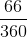, to find the percentage of the base covered.

Statement II gives us the radius of the base, which lets us also know the radius of the sticker; however, this will not be helpful in finding the percentage of the base covered, so Statement II is not really helpful.

Thus, Statement I is sufficient and Statement II is not.

Recap:

Dirk bought a sticker to cover part of the base of his scuba tank. The sticker and the base have the same radius. Given the following, find the percentage of the base that the sticker covers.

I) The central angle of the sticker isII) The base of the tank has a radius of 5 inches

So, according to Statement I, the angle of the sticker is 66 degrees. Because we are dealing with a circle, we can find the percentage of the circle covered by putting 66 over 360.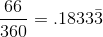The sticker covers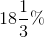of the scuba tank.

### Example Question #2 : Sectors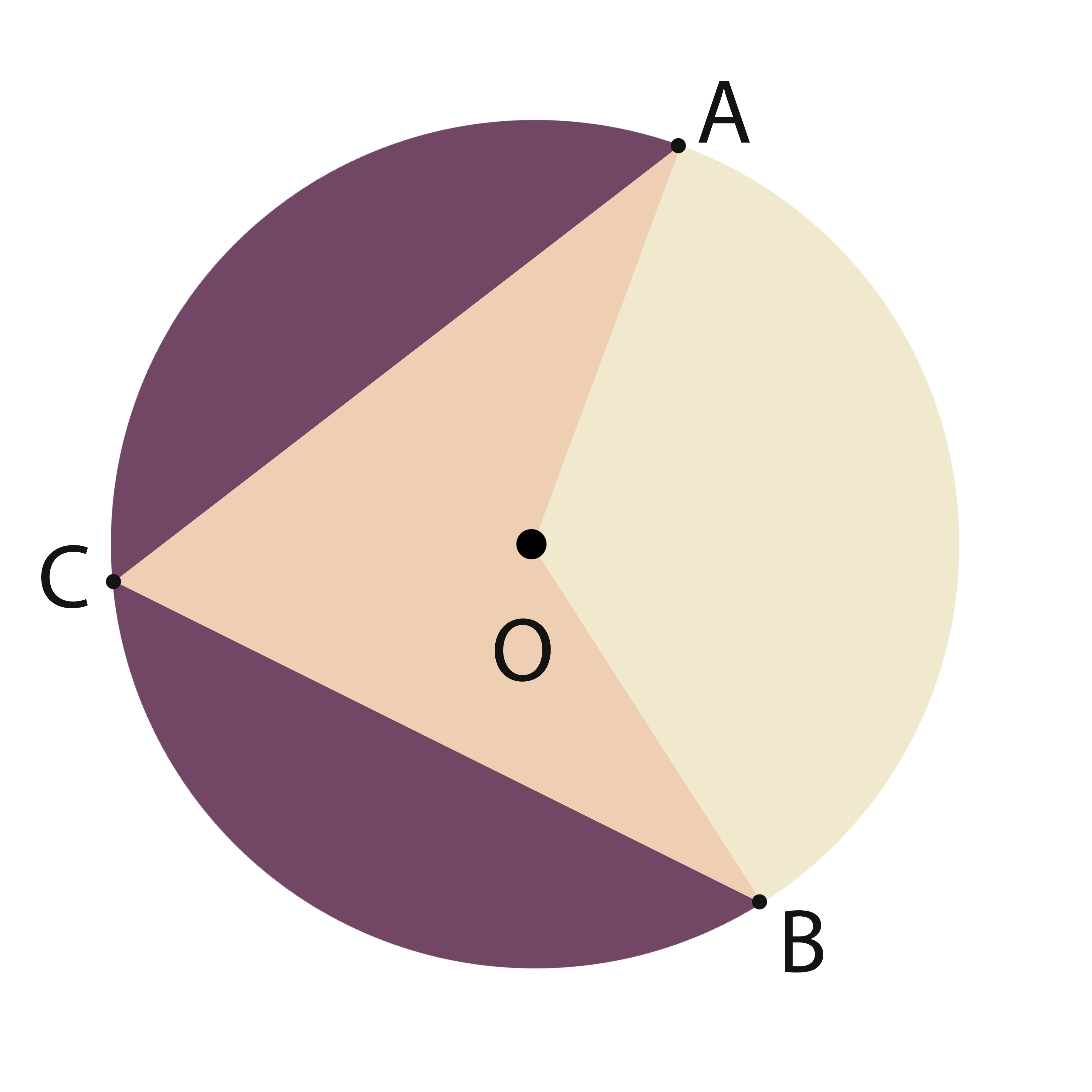What is the area of sector AOB?

1)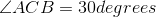2) Arclength of AB is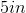Statement 2 alone is sufficient.

Together the two statements are sufficient.

Either of the statements is sufficient.

Statement 1 alone is sufficient.

Neither of the statements, separate or together, is sufficient.

Together the two statements are sufficient.

Explanation:

The angle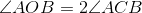, so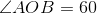.

The Arc length can be related to the radius as follows: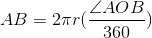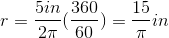From there, the area of the sector can be found: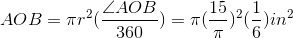### Example Question #8 : Sectors

Consider circle F with sector BFG. What percent of F is BFG?

I) Circle F has a radius of 5 meters and a circumference of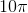meters.

II) Sector BFG has a central angle measure of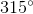.

Either statement is sufficient to answer the question.

Statement I is sufficient to answer the question, but statement II is not sufficient to answer the question.

Statement II is sufficient to answer the question, but statement I is not sufficient to answer the question.

Both statements are needed to answer the question.

Statement II is sufficient to answer the question, but statement I is not sufficient to answer the question.

Explanation:

Consider circle F with sector BFG. What percent of F is BFG?

I) Circle F has a radius of 5 meters and a circumference ofmeters

II) Sector BFG has a central angle measure ofTo find the percentage of a circle covered by a sector, we need to know the central angle. A circle will always have, so if we put the angle given to us over. So, II) allows us to answer the question: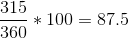So sector BFG is 87.5% of circle F

I) Give us interesting information, but not helpful in regards to the current question. It doesn't matter what the radius is, and the circumference is also irrelevant.

Therefore, II) is sufficient, but I) is not.

### Example Question #9 : Sectors

Jane wants to put a sector-shaped sticker on the bottom of her cylindrical water bottle. The water bottle has a circular base. Find the percent of the base that the sticker covers

I) The sticker has a thickness of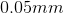.

II) The central angle of the sticker has a measure of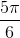.

Statement I is sufficient to answer the question, but statement II is not sufficient to answer the question.

Both statements are needed to answer the question.

Either statement is sufficient to answer the question.

Statement II is sufficient to answer the question, but statement I is not sufficient to answer the question.

Statement II is sufficient to answer the question, but statement I is not sufficient to answer the question.

Explanation:

Jane wants to put a sector-shaped sticker on the bottom of her cylindrical water bottle. The water bottle has a circular base. Find the percent of the base that the sticker covers

I) The sticker has a thickness ofII) The central angle of the sticker has a measure ofI) is irrelevant. The thickness of  the sticker doesn't help us find the area it covers. Statment I) is trying to distract you with 3 dimensions, when we only need to worry about 2.

II) is much more helpful. If we know the central angle, we can find the percentage the sector covers. To find the "answer" perform the following: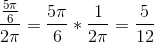So we are dealing with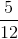of the circle, or 41.67%

### Example Question #10 : Sectors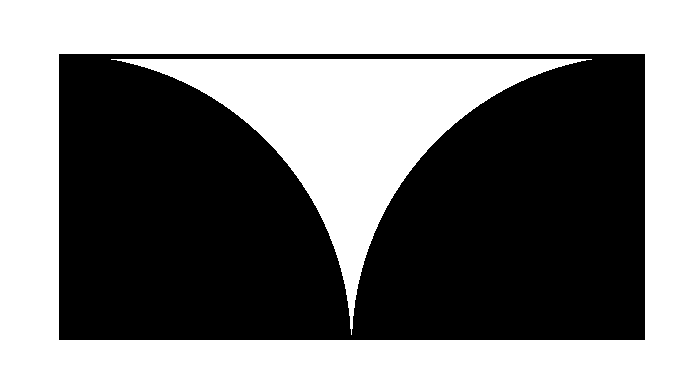The above figure shows two quarter circles inscribed inside a rectangle. What is the total area of the white region?

Statement 1: The area of the black region is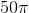square centimeters.

Statement 2: The rectangle has perimeter 60 centimeters.

EITHER statement ALONE is sufficient to answer the question.

BOTH statements TOGETHER are sufficient to answer the question, but NEITHER statement ALONE is sufficient to answer the question.

BOTH statements TOGETHER are insufficient to answer the question.

Statement 1 ALONE is sufficient to answer the question, but Statement 2 ALONE is NOT sufficient to answer the question.

Statement 2 ALONE is sufficient to answer the question, but Statement 1 ALONE is NOT sufficient to answer the question.

EITHER statement ALONE is sufficient to answer the question.

Explanation:

The width of the rectangle is equal to the radius of the quarter circles, which we call; the length is twice that, or.

The area of the rectangle is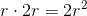; the total area of the two black quarter circles is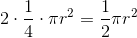, so the area of the white region is their difference,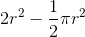Therefore, all that is needed to find the area of the white region is the radius of the quarter circle.

If we know that the area of the black region iscentimeters, then we can deduceusing this equation: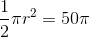If we know that the perimeter of the rectangle is 60 centimeters, we can deducevia the perimeter formula: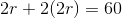Either statement alone allows us to find the radius and, consequently, the area of the white region.

### Example Question #1 : Dsq: Calculating The Area Of A Sector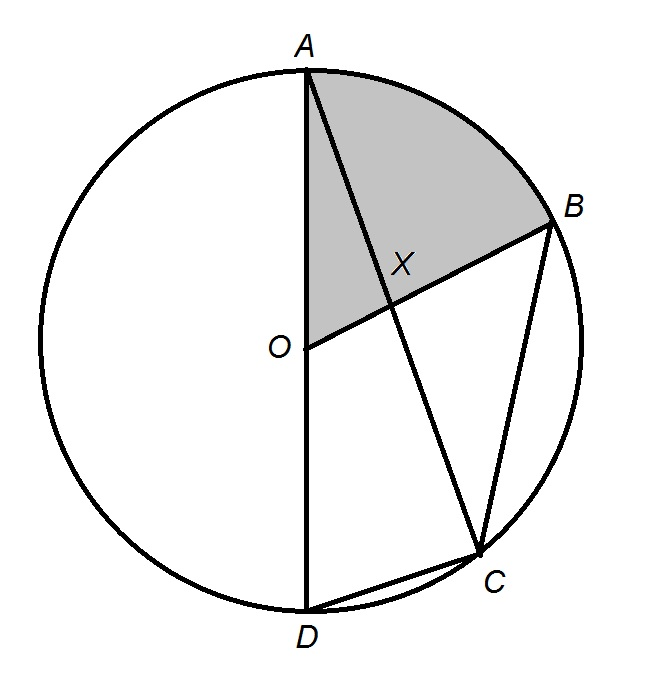The circle in the above diagram has center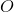. Give the area of the shaded sector.

Statement 1: The circle has circumference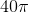.

Statement 2: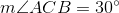BOTH statements TOGETHER are insufficient to answer the question.

BOTH statements TOGETHER are sufficient to answer the question, but NEITHER statement ALONE is sufficient to answer the question.

EITHER statement ALONE is sufficient to answer the question.

Statement 2 ALONE is sufficient to answer the question, but Statement 1 ALONE is NOT sufficient to answer the question.

Statement 1 ALONE is sufficient to answer the question, but Statement 2 ALONE is NOT sufficient to answer the question.

BOTH statements TOGETHER are sufficient to answer the question, but NEITHER statement ALONE is sufficient to answer the question.

Explanation:

To find the area of a sector of a circle, we need a way to find the area of the circle and a way to find the central angle of the sector.

Statement 1 alone gives us the circumference; this can be divided byto yield the radius, and that can be substituted forin the formulato find the area. However, it provides no clue that might yield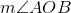.

Statement 2 alone asserts that. This is an inscribed angle that intercepts the arc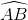; therefore, the arc - and the central angle that intercepts it - has twice this measure, or. Therefore, Statement 2 alone gives the central angle, but does not yield any clues about the area.

Assume both statements are true. The radius is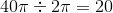and the area is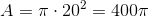. The shaded sector is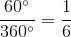of the circle, so the area can be calculated to be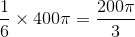.

### Example Question #2 : Dsq: Calculating The Area Of A SectorThe circle in the above diagram has center. Give the ratio of the area of the white sector to that of the shaded sector.

Statement 1:Statement 2: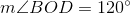BOTH statements TOGETHER are sufficient to answer the question, but NEITHER statement ALONE is sufficient to answer the question.

EITHER statement ALONE is sufficient to answer the question.

Statement 1 ALONE is sufficient to answer the question, but Statement 2 ALONE is NOT sufficient to answer the question.

BOTH statements TOGETHER are insufficient to answer the question.

Statement 2 ALONE is sufficient to answer the question, but Statement 1 ALONE is NOT sufficient to answer the question.

EITHER statement ALONE is sufficient to answer the question.

Explanation:

Statement 1 alone asserts that. This is an inscribed angle that intercepts the arc; therefore, the arc - and the central angle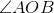that intercepts it - has twice this measure, orStatement 2 alone asserts that. By angle addition,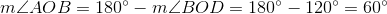Either statement alone tells us that the shaded sector isof the circle, and that the white sector is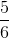of it; it can be subsequently calculated that the ratio of the areas is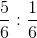, or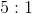.

### All GMAT Math Resources